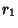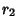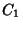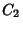The Locus of points of equal Power with respect to two nonconcentric Circles which is Perpendicular to the line of centers (the Chordal Theorem; Dörrie 1965). Let the circles have Radiiandand their centers be separated by a distance. If the Circles intersect in two points, then the radical line is the line passing through the points of intersection. If not, then draw any two Circles which cut each original Circle twice. Draw lines through each pair of points of intersection of each Circle. The line connecting their two points of intersection is then the radical line.

The radical line is located at distances(1)(2)

along the line of centers fromand, respectively, where(3)

The radical line of any two Polar Circles is the Altitude from the third vertex.

References

Coxeter, H. S. M. and Greitzer, S. L. Geometry Revisited. Washington, DC: Math. Assoc. Amer., pp. 31-34, 1967.

Dixon, R. Mathographics. New York: Dover, p. 68, 1991.

Dörrie, H. 100 Great Problems of Elementary Mathematics: Their History and Solutions. New York: Dover, p. 153, 1965.

Johnson, R. A. Modern Geometry: An Elementary Treatise on the Geometry of the Triangle and the Circle. Boston, MA: Houghton Mifflin, pp. 28-34 and 176-177, 1929.